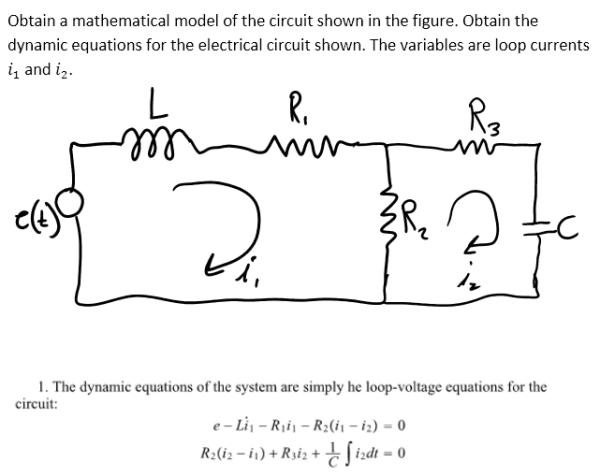Obtain a mathematical model of the circuit shown in the figure. Obtain the dynamic equations for the electrical circuit shown in the figure. The variables are loop currents i1 and i2.Obtain a mathematical model of the circuit shown in the figure. Obtain the dynamic equations for the electrical circuit shown in the figure. The variables are loop currents i1 and i2.

System Dynamics Page 2 dynamics dynamics dynamics dynamics dynamics dynamics dynamics System dynamics Page 3